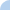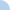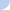Honors Geometry: Easy Quiz on Coordinate Geometry Basics!

You can take this quiz as many times as you would like. Every answer involving a square root should be simplified. There are 15 questions.

 This quiz is timed. The total time allowed for this quiz is 4 hours.Quiz Log In